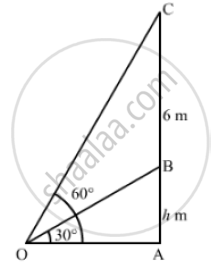# The Vertical Tower Stands on a Horizontal Plane and is Surmounted by a Vertical Flagstaff of Height 6m.T of the Top of the Flagstaff 60 . - Mathematics

The vertical tower stands on a horizontal plane and is surmounted by a vertical flagstaff of height 6m. At a point on the plane, the angle of elevation of the bottom of the flagstaff is
30 and that of the top of the flagstaff 60 . Find the height of the tower
[Use sqrt(3) 1.732 ]

#### SolutionLet AB be the tower and BC be the flagstaff,
We have,
BC = 6m, ∠AOB = 30° and ∠AOC - 60°
Let AB = h
In  ΔAOB

tan 30° = (AB)/(OA)

⇒ 1/ sqrt(3) = h/( OA)

 ⇒  OA = h sqrt(3)                ..............(i)

Now in Δ AOC,

 tan 60° = (AC)/(OA)

⇒   sqrt(3) = (AB +BC)/ (h sqrt(3))               [ Using (i)]

⇒ 3h = h + 6

⇒ 3h  - h = 6

⇒ 2h = 6

⇒  h= 6/2

⇒  h = 3m

So, the height of the tower is 3 m.

Concept: Heights and Distances
Is there an error in this question or solution?
Chapter 14: Height and Distance - Exercises

#### APPEARS IN

RS Aggarwal Secondary School Class 10 Maths
Chapter 14 Height and Distance
Exercises | Q 7

Share